# Deep Learning Course Project: Japanese hostel price prediction.

in hive-175254 •  7 days ago  (edited)

Hostels are very important for backpackers and teens because they provide an economical housing option and promote travel. When we travel, we meet new people, engage in meaningful conversation, and widen our horizon of knowledge. So, I decided to train a model to predict the hostel prices in Japan.

``````def customize_dataset(dataframe_raw):
dataframe = dataframe_raw.copy(deep=True)
# drop some columns
dataframe = dataframe.drop(['Unnamed: 0', 'hostel.name', 'Distance', 'lon', 'lat', 'atmosphere', 'cleanliness',
'facilities', 'location.y', 'security', 'staff'], axis=1)
#     for col in ['City', 'rating.band']:
#        # normalizing incoming data
#        dataframe[col] = (dataframe[col] - min(dataframe[col])) / (max(dataframe[col]) - min(dataframe[col]))

# dropping any row that contains at least on missing value
dataframe = dataframe.dropna(axis=0)

return dataframe

dataframe = customize_dataset(dataframe)

``````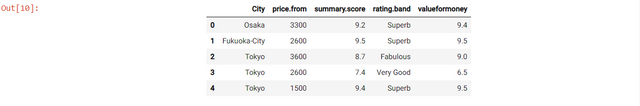My main objective was to concentrate on four important factors which determine hostel prices. These factors were location, value for money, customer rating and the rating range. Once these factors were correlated, they could be used for predictions. Hence, finding the factors which could correlate with each other was very important.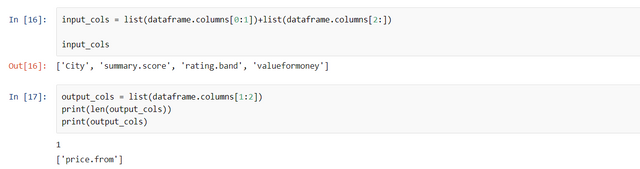``````# Convert from Pandas dataframe to numpy arrays

def dataframe_to_arrays(dataframe):
# Make a copy of the original dataframe
dataframe1 = dataframe.copy(deep=True)
# Convert non-numeric categorical columns to numbers
for col in categorical_cols:
dataframe1[col] = dataframe1[col].astype('category').cat.codes

# Extract input & outupts as numpy arrays
inputs_array = dataframe1[input_cols].to_numpy()
targets_array = dataframe1[output_cols].to_numpy()
return inputs_array, targets_array

inputs_array, targets_array = dataframe_to_arrays(dataframe)
inputs_array, targets_array

``````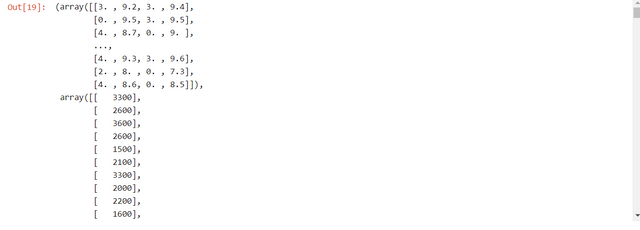``````inputs = torch.from_numpy(inputs_array).type(torch.float32)
targets = torch.from_numpy(targets_array).type(torch.float32)

dataset = TensorDataset(inputs, targets)

val_percent = 0.1 # between 0.1 and 0.2
val_size = int(num_rows * val_percent)
train_size = num_rows - val_size

# Use the random_split function to split dataset into 2 parts of the desired length
train_ds, val_ds = random_split(dataset, [train_size, val_size])

input_size = len(input_cols)
output_size = len(output_cols)
print(len(input_cols))
print(len(output_cols))

``````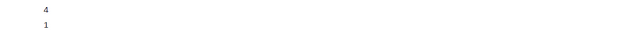``````class HousingModel(nn.Module):
def __init__(self):
super().__init__()
self.linear1 = nn.Linear(input_size, 8)
self.linear2 = nn.Linear(8, 16)
self.linear3 = nn.Linear(16, output_size)

def forward(self, xb):
out = self.linear1(xb)
out = F.relu(out)
out = self.linear2(out)
out = F.relu(out)
out = self.linear3(out)
return out

def training_step(self, batch):
inputs, targets = batch
out = self(inputs)                 # Generate predictions
loss = F.l1_loss(out, targets)    # Calculate loss
return loss

def validation_step(self, batch):
inputs, targets = batch
out = self(inputs)                 # Generate predictions
loss = F.l1_loss(out, targets)    # Calculate loss
return {'val_loss': loss.detach()}

def validation_epoch_end(self, outputs):
batch_losses = [x['val_loss'] for x in outputs]
epoch_loss = torch.stack(batch_losses).mean()   # Combine losses
return {'val_loss': epoch_loss.item()}

def epoch_end(self, epoch, result):
print("Epoch [{}], val_loss: {:.4f}".format(epoch, result['val_loss']))

model = HousingModel()
outputs = [model.validation_step(batch) for batch in val_loader]
return model.validation_epoch_end(outputs)

history = []
optimizer = opt_func(model.parameters(), max_lr, weight_decay=weight_decay)
sched = torch.optim.lr_scheduler.OneCycleLR(optimizer, max_lr, epochs=epochs,
for epoch in range(epochs):
# Training Phase
loss = model.training_step(batch)
loss.backward()
optimizer.step()
# Validation phase
model.epoch_end(epoch, result)
history.append(result)
return history

epochs = 40
max_lr = 1.5
weight_decay = 1e-8

history
``````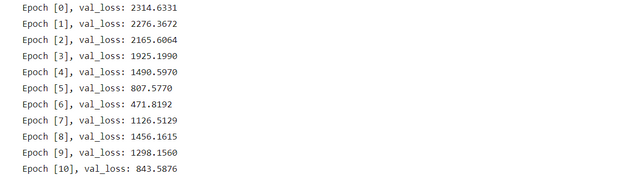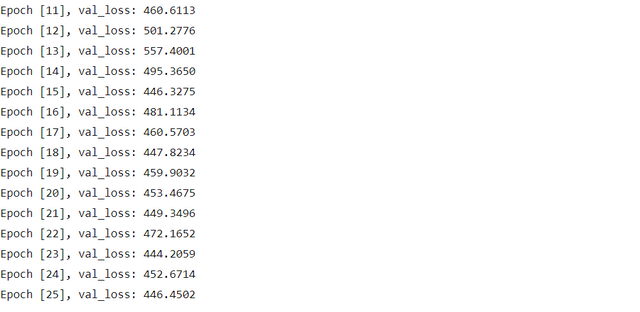This principle of correlation is commonly used by travel websites/apps to help customers find the right hostel by running price predicting algorithms.

``````losses = [r['val_loss'] for r in [result] + history]
plt.plot(losses, '-x')
plt.xlabel('epoch')
plt.ylabel('val_loss')
plt.title('val_loss vs. epochs');

``````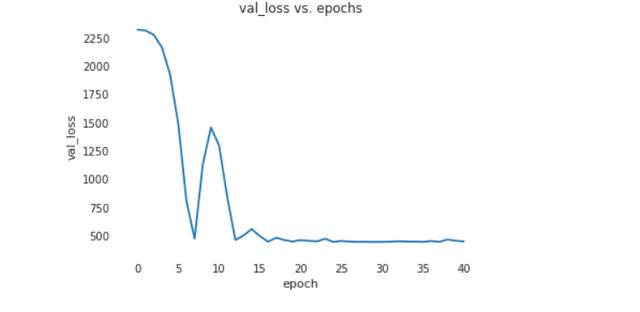``````def predict_single(x, model):
xb = x.unsqueeze(0)
return model(x).item()
``````
``````x, target = val_ds
pred = predict_single(x, model)
print("Input: ", x)
print("Target: ", target.item())
print("Prediction:", pred)
``````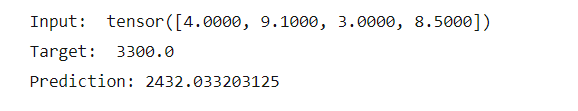``````x, target = val_ds
pred = predict_single(x, model)
print("Input: ", x)
print("Target: ", target.item())
print("Prediction:", pred)

``````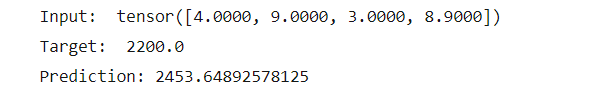According to my algorithm, the predictions were close to the actual price. Hence, to code a good algorithm our data should have an equal number of hostels from different price segments.